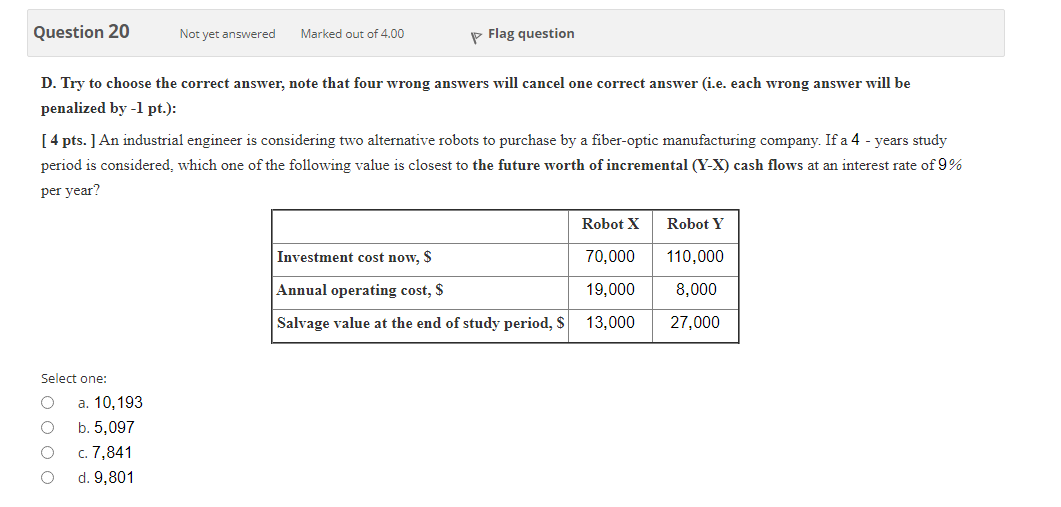Create an Account

Home / Questions / Try to choose the correct answer, note that four wrong answers will cancel one correct ans...

Try to choose the correct answer, note that four wrong answers will cancel one correct answer (i.e. each wrong answer will be penalized by -1 pt.): [ 4 pts. ] An industrial engineer is considering

Try to choose the correct answer, note that four wrong answers will cancel one correct answer (i.e. each wrong answer will be penalized by -1 pt.): [ 4 pts. ] An industrial engineer is considering two alternative robots to purchase by a fiber optic manufacturing company. If a 4 - years study period is considered, which one of the following value is closest the future worth of incremental (Y-X) cash flows at an interest rate of 9% per year? Robot X Robot Y Investment cost now, \$ 70,000 19,000 110,000 8,000 Annual operating cost, \$ Salvage value at the end of study period, \$ 13,000 27,000 Select one: a. 10,193 O b. 5,097 c. 7,841 d. 9,801May 05 2021 View more View LessSubscribe To Get Solution